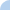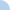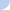Surface Area & Volume of Cylinders

Include units of measure with all of your answers.

If your answer is in linear units you should show units of measure in one of the following ways...
- ft
- in

If your answer is in square units you should show units of measure in one of the following ways...
- in^2
- ft^2

If your answer is in cubic units you should show units of measure in one of the following ways...
-in^3
- ft^3Quiz Log In